• 针对非线性非高斯系统的状态估计问题，提出一种新的高精度自适应粒子滤波算法．该算法采用有限差分扩展卡尔曼滤波器产生优选的建议分布函数，融入最新量测信息，有效克服了粒子退化问题；考虑到预测误差对粒子采样...
• 引入概率回退的方法对机器人的初始状态进行估计，采用窗口滤波更新粒子集合，根据对机器人位置估计的情况动态更新粒子集合的大小，得到一种改进的粒子滤波算法-稳健的自适应粒子滤波算法。仿真结果表明：该算法充分...
• 针对量测不确定条件下多传感器量测数据的有效利用问题, 提出一种多传感器自适应粒子滤波算法. 利用 随机采样策略和量测模型转移概率实现当前时刻多传感器量测集合的采样, 通过粒子滤波中重采样步骤完成估计状 ...
• 针对粒子滤波（Particle filter）算法的粒子衰退和计算量过大问题，提出一种将P－N跟踪器与粒子滤波算法结合的目标跟踪方法。首先构造P－N跟踪器，利用跟踪器来确定目标区域范围并输出置信度，以此作为对目标物体...
• 针对粒子滤波存在计算效率低和因粒子贫化导致的计算精度下降问题,基于KLD(Kullback Leibler distance)采样和蝙蝠算法,提出一种可动态调整粒子规模的自适应粒子滤波算法.首先,在重要性采样中利用KLD采样动态调整粒子...
• 针对粒子滤波算法时间复杂度高的问题,引入一种在滤波过程中粒子数可以根据过程噪声方差大小进行调整的自适应粒子滤波算法,即KLD-Sampling粒子滤波算法。该算法在保证一定滤波精度的前提下,可以有效地减少滤波过程中...
•研究论文
•研究论文
• 自适应粒子滤波：如何计算粒子数 在传统的基于图像的目标跟踪和检测算法中，粒子滤波经常被提到。但是，翻各种资料的时候，大段大段的公式，让人根本看不下去。其实，粒子滤波的基本思想还是很直接明了的，但是非常...
自适应粒子滤波：如何计算粒子数
在传统的基于图像的目标跟踪和检测算法中，粒子滤波经常被提到。但是，翻各种资料的时候，大段大段的公式，让人根本看不下去。其实，粒子滤波的基本思想还是很直接明了的，但是非常有用。下面推荐一个自己认为写得不错的内容，作为入门。
怎样从实际场景上理解粒子滤波（Particle Filter）
下面评论里边有代码和例程，可以一块学习。
下面博客的内容，打算接着上面那个基本的粒子滤波思想，写一下如何自适应计算滤波的粒子个数。主要参考论文如下：
Adapting the Sample Size in Particle Filters Through KLD-Sampling

如何自适应地计算粒子数呢？
大家都知道，粒子滤波是用粒子来近似后验分布，也就是说粒子数越多的区域，那么真实状态落在这个区域的概率就越大。换句话说，越多的采样粒子数，那么是不是就能更好地反映真实情况呢？答案是这样的。
但是，现在存在一个问题：究竟在实际的场景中需要多少的粒子才认为反映出了真实情况？这里用一个计算公式来计算估计出的概率分布 p() 和真实概率分布 q() 之间的距离，也就是KL-distance。

现在有衡量两个概率密度分布之间的距离公式啦，后面只需要用这个公式计算我们得到的概率分布和真实分布就可以啦。
等等，真实分布如何得到啊。。嗯，那是不可能得到的。
好，接下来开始是作者如何用各种办法来计算这个距离的，因为真实分布根本得不到呀。
各种假设：假设现在有n个粒子，分别来自于k个不同的bin（翻译成箱子？有点像图像梯度直方图里边那个），而且每一个bin里边分别有个粒子。当然，所有bin里边的粒子数加起来肯定等于n。相当于把n个粒子分别放到k个不同的bin里边去。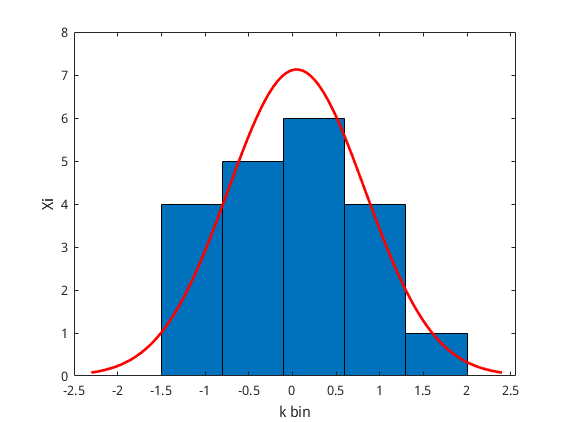稍微画了个图。假设上图的红色线条是一维概率密度函数，我们用蓝色的直方图去近似这个曲线。现在图中一共有5个bin（5个蓝色条），每个bin里边放的粒子数为对应的纵坐标。一个直观的感受是：bin的数目越多，则可以更好地近似这条曲线。
还是假设：假设每一个bin的真实概率分布为,而此时由我们采样出来的概率为.那么每一个是怎么计算的呢？很简单，这个bin里的粒子数目除以总数n.

统计数据的似然比就可以按下面的公式计算啦。

可以把Xj替换啦，一定要变形成为第一个计算距离的公式(1)，所以得到下面

按公式(1)整理得到如下：

不知道这个公式怎么居中。。就这样子吧。这里，虽然不知道真实的概率分布p，但是他们之间的距离已经可以用等式左边的来替代啦。
根据论文J.A. Rice. Mathematical Statistics and Data Analysis. Duxbury Press, second edition, 1995.可以得到

哈哈，公式(3) 等式左边是满足这个卡方分布的。（链接是wiki的资料）
重点来了！！
我们现在只需要期待两个概率之间的距离小于某一阈值就可以啦。好，那么，我们构建概率来表达。

根据卡方分布的性质，明显有以下等式（这个具体去看卡方分布的性质吧）

结合公式（4）来看，只要就可以满足等式（5）啦，等式（5）可以由查表知道此时的概率是多少。

公式6又怎么计算呢，很好。有论文给出了近似计算等式。这个公式我截图算了，有点难打。如下：

举个例子，当后面的时，大概公式（5）计算出来的概率为98%。
也就是说我们采样出来的概率分布置信度为98%.
以上就是如何计算自适应粒子个数的全部推到导啦。公式7中的k可以用收集直方图或者用kd-tree的方式得到。
主要的思想就是用卡方分布去近似计算估计概率分布和真实概率分布之间的距离。
以上。
扔一个论文里边的算法流程，下面按这个流程编写自适应算法。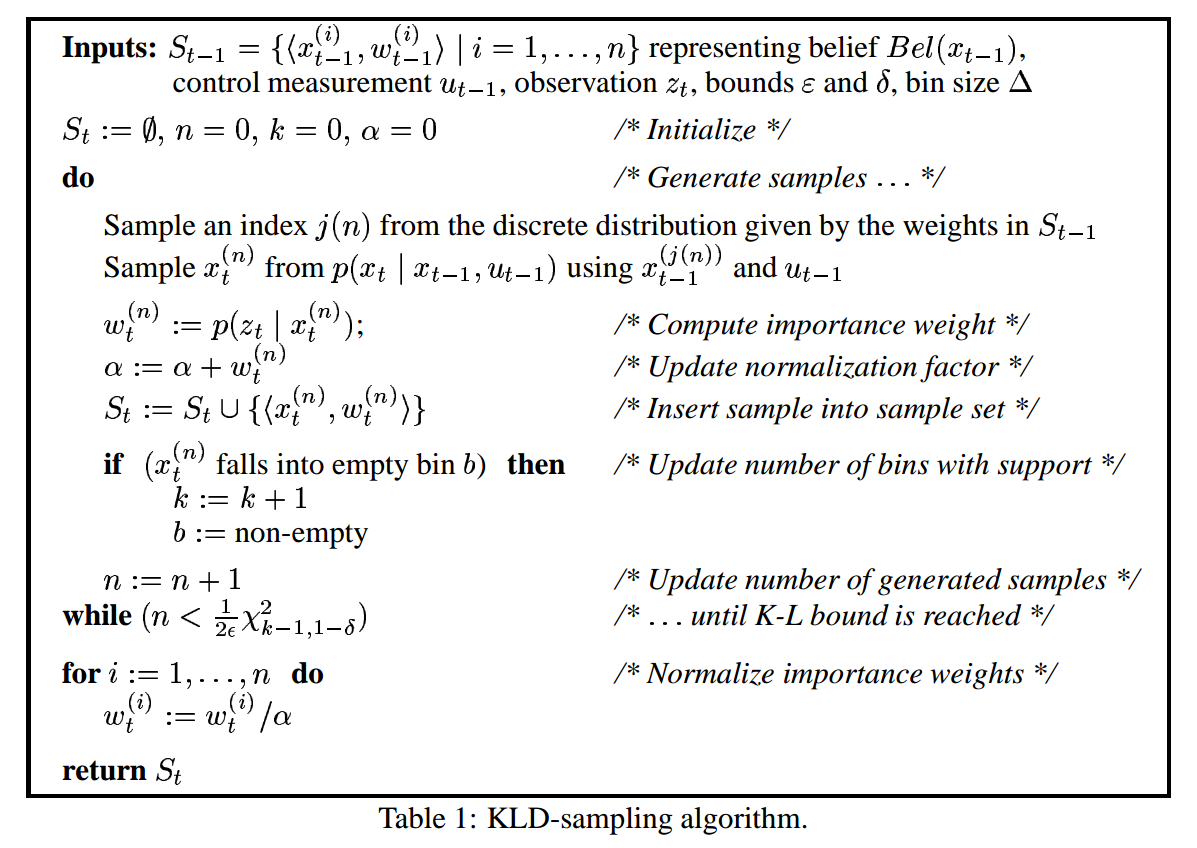接下来是实现部分啦，打算采用C++编程实现基本粒子滤波和自适应粒子数的计算。
等待更新...

Update  2018/9/3
把之前的代码整理了一下，现在更新一下代码具体怎么实现。基本代码是基于Rob Hess源码，具体分析看下面这篇博客。
https://blog.csdn.net/hujingshuang/article/details/45535423
整个工程需要完成这样一个任务，在视频的第一幅图像上，用鼠标画一个矩阵框，然后用粒子滤波算法跟踪矩形框内的目标。
基本思想：
1）提取初始矩形框内目标的特征信息，这里直接采用颜色直方图来表示。
将图像BGR空间转换到HSV空间。提取矩形框内颜色直方图。归一化。
2）提取每个粒子所在位置的颜色直方图，归一化后与1）中目标信息计算距离，距离越小认为目标相似度高，权重大。
3）每次根据权重调整粒子数目的分布，简而言之，权重越大的地方认为目标出现的可能性越大，那就在附近多放一些粒子。（SIR 思想）
最简单的就是直接在该位置按高斯分布放置就行。new_x=x+normal_distribution(0.0,variance)new_y=y+normal_distribution(0.0,variance)
4）每次放完粒子之后，计算它的权重，把权重最大的粒子画出来，认为找到了目标。
5）循环以上过程。
好了，基本的这个流程就是这样子。
首先，定义基本粒子如下：
struct histogram
{
float histo[NH*NS + NV];   /**< histogram array */
int n;                     /**< length of histogram array */
};

struct particle {
float x;          /**< current x coordinate */
float y;          /**< current y coordinate */
float s;          /**< scale */
float xp;         /**< previous x coordinate */
float yp;         /**< previous y coordinate */
float sp;         /**< previous scale */
float x0;         /**< original x coordinate */
float y0;         /**< original y coordinate */
int width;        /**< original width of region described by particle */
int height;       /**< original height of region described by particle */
shared_ptr<histogram> hist; /**< reference histogram describing region being tracked */
float w;          /**< weight */
};
解释一下，struct histogram 表示提取的直方图，很简单，就是一个浮点数组，n=NH*NS + NV表示数组大小。
每个粒子particle拥有很多信息，当前的图像坐标x，y，先前的坐标xp，yp，矩形框信息width，height，每个框的缩放比例s，当然还有这个矩形框提取得到颜色直方图啦，放在指针 hist中。
现在我们用鼠标提取第一帧图像的目标如下：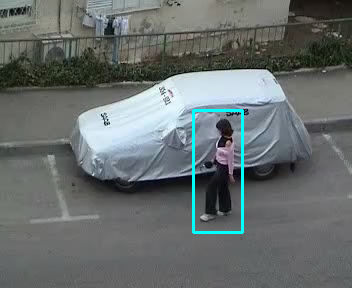然后，得到要跟踪的目标信息，将这些信息放在minitParticle中，初始化粒子如下：
void adaptivePF::init_distribution()
{
float normW=1.0/mN;
float  x=minitParticle->x0;
float y=minitParticle->y0;

vec_w.resize(mN);
//init_distribution
for(int i=0;i<mN;i++)
{

mParticles[i].x0=x;
mParticles[i].xp=x;
mParticles[i].x=x;
mParticles[i].y0=y;
mParticles[i].yp=y;
mParticles[i].y=y;
mParticles[i].sp=1.0;
mParticles[i].s=1.0;
mParticles[i].height=minitParticle->height;
mParticles[i].width=minitParticle->width;
mParticles[i].hist=shared_ptr<histogram>(new histogram);
mParticles[i].w=normW;
mCDF[i]=i*normW;
//mParticles_tmp
mParticles_tmp[i].hist=shared_ptr<histogram>(new histogram);
vec_w[i]=mParticles[i].w;
}
mCDF[mN]=1.0;
}
所有粒子都初始化为初始位置。然后决定下一帧图像到来时，这些粒子应该怎么运动呢，也就是action model，如下：
void adaptivePF::transition(int p_idx, int new_size)
{
float x, y, s;
// sample new state using second-order autoregressive dynamics

s=A1 * ( mParticles[p_idx].s - 1.0 ) + A2 * ( mParticles[p_idx].sp - 1.0 ) +B0 * Gaussian_s(generator) + 1.0;
x=mParticles[p_idx].x+Gaussian_x(generator);
y=mParticles[p_idx].y+Gaussian_y(generator);

mParticles_tmp[new_size].x=MAX(1.0,MIN(float(mWidth-1.0),x));
mParticles_tmp[new_size].y=MAX(1.0,MIN(float(mHeight-1.0),y));
mParticles_tmp[new_size].s=MAX(0.1,s);

mParticles_tmp[new_size].xp=mParticles[p_idx].x;
mParticles_tmp[new_size].yp=mParticles[p_idx].y;
mParticles_tmp[new_size].sp=mParticles[p_idx].s;
mParticles_tmp[new_size].x0=mParticles[p_idx].x0;
mParticles_tmp[new_size].y0=mParticles[p_idx].y0;
mParticles_tmp[new_size].w=mParticles[p_idx].w;

gsl_vector_set(bin_temp,0,mParticles_tmp[new_size].x);
gsl_vector_set(bin_temp,1,mParticles_tmp[new_size].y);
}
很简单，直接在上一次位置处叠加高斯分布得到新位置即可。然后在新位置使用sensor model，检测该处的颜色直方图。
float adaptivePF::evaluate(int new_size)
{
calc_histogram(mParticles_tmp[new_size]);
normalize_histogram(mParticles_tmp[new_size]);
mParticles_tmp[new_size].w=histo_dist_sq(mParticles_tmp[new_size].hist,ref_histo);
vec_w[new_size]=mParticles_tmp[new_size].w;
return mParticles_tmp[new_size].w;
}
int adaptivePF::histo_bin(float h, float  s, float  v)
{
int hd, sd, vd;
s=s/255.0;
v=v/255.0;
/* if S or V is less than its threshold, return a "colorless" bin */
vd = MIN( (int)(v * NV / V_MAX), NV-1 );
if( (s) < S_THRESH  ||  v < V_THRESH )
return NH * NS + vd;

/* otherwise determine "colorful" bin */
hd = MIN( (int)(h * NH / H_MAX), NH-1 );
sd = MIN( (int)(s * NS / S_MAX), NS-1 );
return sd * NH + hd;

}
{
int sum=0;
int n=mm.hist->n;
for(int i=0;i<n;i++)
{
sum+=mm.hist->histo[i];
}
float inv_sum=1.0/sum;
for(int i=0;i<n;i++)
{
mm.hist->histo[i] *=inv_sum;
}

}
{
/*
According the the Battacharyya similarity coefficient,

D = \sqrt{ 1 - \sum_1^n{ \sqrt{ h_1(i) * h_2(i) } } }
*/
float sum = 0;
int n = h1->n;
for(int  i = 0; i < n; i++ )
{
sum += sqrt( h1->histo[i]* h2->histo[i]);
}
return std::exp( -LAMBDA *(1.0-sum) );
}
粒子滤波最重要的两个过程：action model和sensor model就是这样。

下面是自适应部分。
直接放代码：
void adaptivePF::update()
{
int p_idx;
//Initialize kd-tree
kd_tree->reset();
mWeightSum=0;
int new_size = 0;
do{
p_idx = SIR();
transition(p_idx,new_size);
mWeightSum+=evaluate(new_size);
kd_tree->insert(bin_temp);
new_size++;
}while(new_size < min_num ||(new_size < max_num && new_size < proper_size(kd_tree->size())));
mN=new_size;

mCDF=0.0;
float invW=1.0/mWeightSum;
for(int i=0;i<mN;i++)
{
mParticles_tmp[i].w=mParticles_tmp[i].w*invW;
mCDF[i+1]=mCDF[i]+mParticles_tmp[i].w;
}
//swap data
particle* tmp=mParticles;
mParticles=mParticles_tmp;
mParticles_tmp=tmp;

}
while(new_size < min_num ||(new_size < max_num && new_size < proper_size(kd_tree->size())));
do-while这个循环控制了每次采样的粒子数目，其中kd_tree->size()就是我们公式 (7)中的k，稍后解释一下这个k怎么得到的。

函数 proper_size() 完成了公式(7)的计算。
float err_bound=0.02;
float conf_quantile=2.0537489;
{
double t0 = 2.0f / (9 * (k-1));
double t1 = 1.0 - t0 + sqrt(t0) * conf_quantile;
return int((k-1)/(2*err_bound) * t1*t1*t1);
}
变量err_bound就是公式里边的，给定bin的大小k，就能根据公式（7）计算得到最合适的粒子数n，然后每次更新通过n来控制下次的粒子采样数目，就可以达到自适应粒子数的目的。
解释下这个k值怎么得到，比较简单，其实就是一个二维直方图（x，y）。这里，我用比较简单的二叉树直接实现了KD-tree。
思想：
粒子坐标x/10,y/10，建立kd-tree。10表示每个bin的间隔是10，也就是0-9的数落在一个bin里，10-19落在另一个。然后kd_size就是bin的数目。
这种方法得到k值，一个直观的感受：
当采样粒子在图像中很分散，则采样粒子数目增加。当采用粒子在图像只收敛到一起（集中），则减少采样粒子数目。
很好理解，当粒子分散时，需要更多的粒子在图像中寻找目标，当粒子收敛时，已经找到目标，减少粒子数目。
下面贴几张过程图像：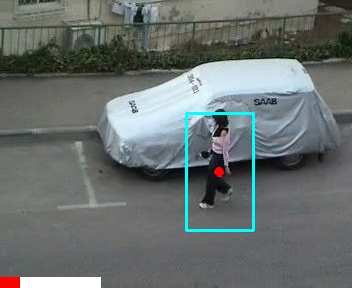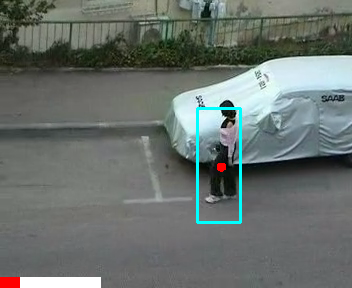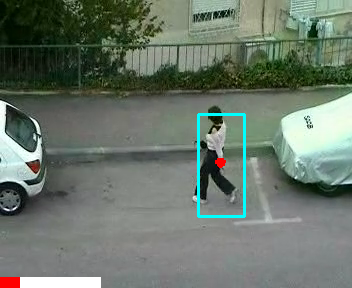可以看到，红色的粒子基本都收敛到一起，下面的bar表示粒子的数目，最大设置为5000，最小是1000.
三张图像粒子个数基本没有变化。
为了说明粒子数目变化，手动增大action model方差，得到下面的图像。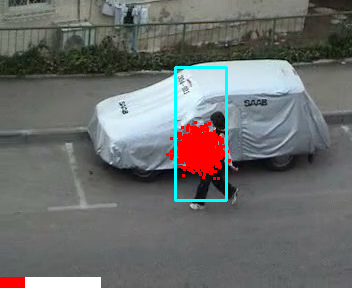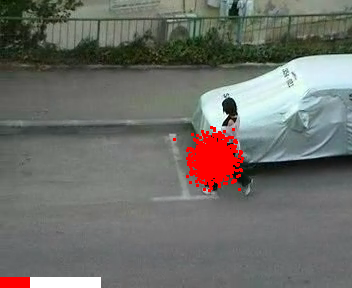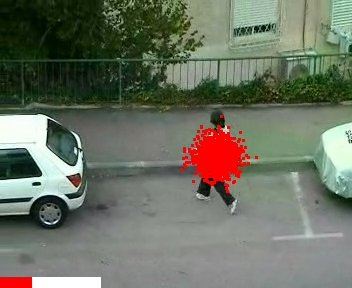OK，这个自己挖的坑，终于填上了。
欢迎留言交流，如果觉得有帮助，点个赞呗。
展开全文目标跟踪
• 进而对局部模型用自适应粒子滤波算法进行近似推理，并以粒子的因式积形式近似系统的状态信度。实验结果表明，该算法能很好地兼顾推理精度和推理时间，其性能优于普通PF算法；与APF算法相比，在不增加推理误差的情况...动态贝叶斯网络 粒子滤波
•研究论文
• 粒子滤波定位代码思想 杭州电子科技大学-自动化学院-智能机器人实验室-Jolen Xie 文章目录粒子滤波定位代码思想1. 代码结构2. 核心代码解读2.1 localizer.cc解读2.1.1 运动更新代码解读void motionUpdate(const ...
自适应粒子滤波定位(AMCL)代码思想
杭州电子科技大学-自动化学院-智能机器人实验室-Jolen Xie

文章目录
自适应粒子滤波定位(AMCL)代码思想1. 代码结构2. 核心代码解读2.1 localizer.cc解读2.1.1 运动更新代码解读void motionUpdate(const Pose2d& odom);2.1.2 测量更新代码解读 void measurementUpdate(const sensor_msgs::LaserScanConstPtr& scan );2.1.3 重采样代码解读 void reSample();
2.2 measurement_model.cc代码解读2.3 pf_localization_node.cc代码解读
3. 运行自己地图需要修改的部分3.1 参数配置文件3.2 pf_localization.launch文件配置

1. 代码结构
整个文件一共有7个头文件：
grid_map.h、localizer.h、measurement_model.h、motion_model.h、particle.h、Pose2d.h、robot.h
有4个源文件：
grid_map.cc、localizer.cc、measurement_model.cc、motion_model.cc
还有一个主文件：
pf_localization_node.cc
代码框架如下图：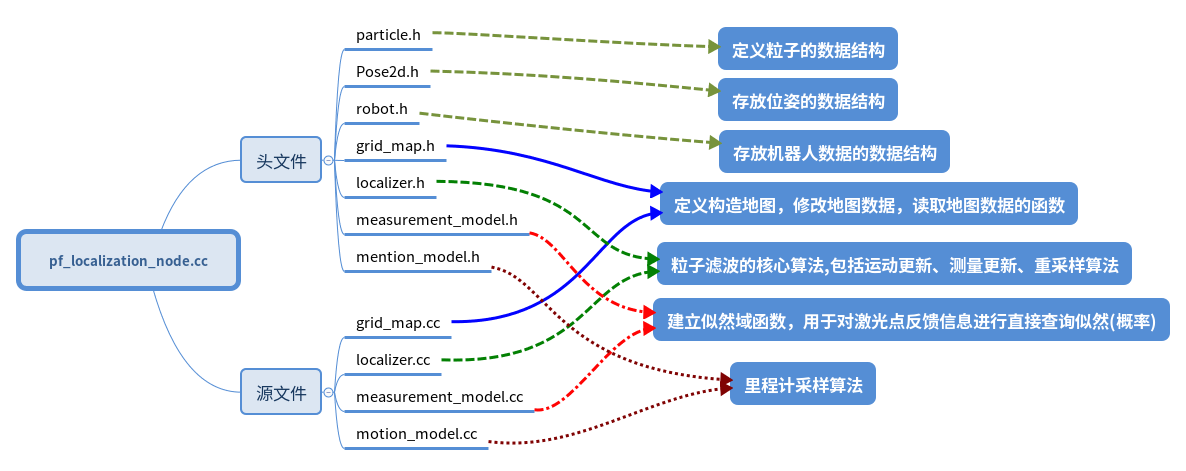2. 核心代码解读
2.1 localizer.cc解读
先看看头文件含有哪些函数
class Localizer{
public:
/* 初始化粒子，在地图可行区域随机撒粒子 */
Localizer(Robot* robot, GridMap* map, MotionModel* motion_model, MeasurementModel* measurement_model, size_t nParticles);

/* 通过odom信息进行运动更新 */
void motionUpdate(const Pose2d& odom);

/* 通过雷达激光信息进行测量更新 */
void measurementUpdate(const sensor_msgs::LaserScanConstPtr& scan );

/* 粒子重采样（重要性采样） */
void reSample();

/* 把粒子姿态信息转化为ros下话题类型geometry_msgs::PoseArray */
void particles2RosPoseArray(geometry_msgs::PoseArray& pose_array);

/* 清除走出地图的粒子以及对粒子权重进行归一化 */
void normalization();
}; //class Localizer

2.1.1 运动更新代码解读void motionUpdate(const Pose2d& odom);
首先先看看中文版概率机器人 P103 中 程序5.6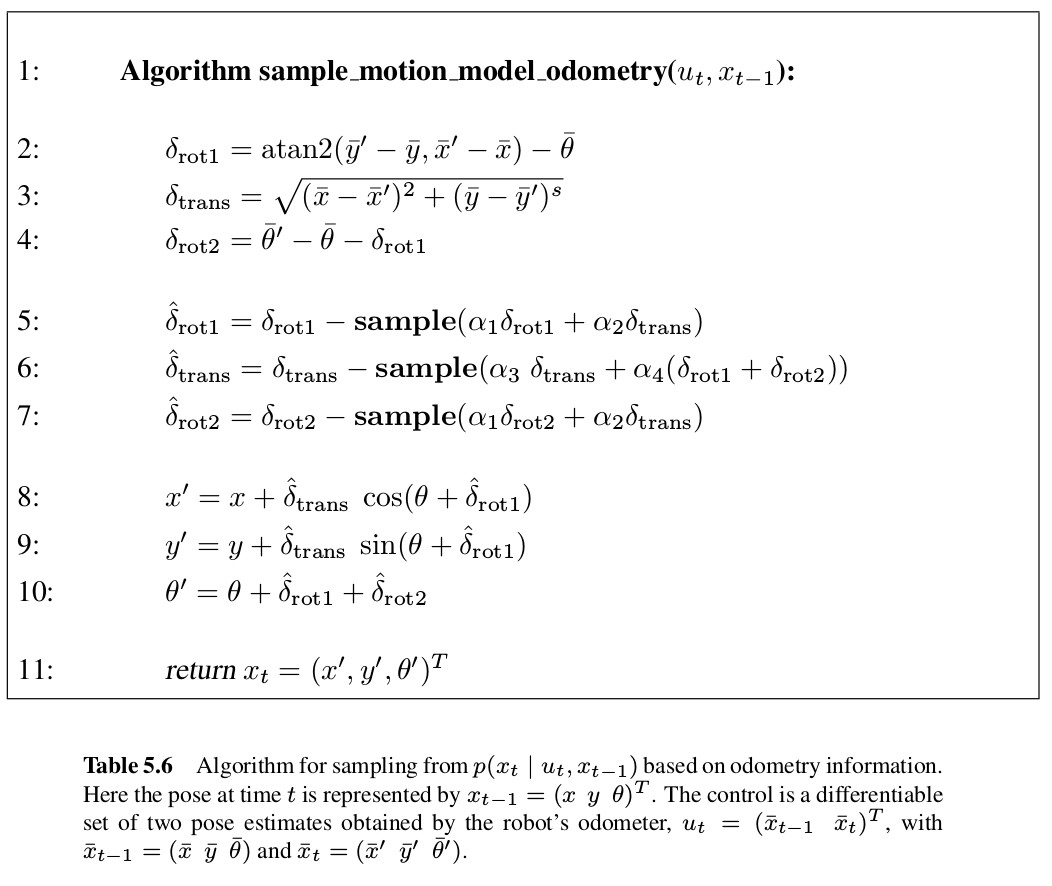从程序5.6中可以看出输入

u

t

u_t

是机器人里程计信息。
算法第2、3行计算了采样时间内运行距离算得的角度差、运行的距离。
第4行代码计算的是里程计测量的角度差和里程计测得位置计算的角度差之间的误差。
算法5-7行在角度、距离和误差上进行高斯采样，该采样基于角度差、运行距离和误差。可以看出走的距离越远，累计误差越大，方差会越大。
算法第8-9行更据单位时间内的移动求累计运行距离和角度。
其中sample(x)是生成均值为0,方差为x的随机样本的函数。
运动更新代码：
void Localizer::motionUpdate ( const Pose2d& odom )//获取odom信息进行运动更新
{
const double MIN_DIST = 0.02; // TODO param 如果运动太近的话就不更新了，防止出现角度计算错误
if ( !is_init_ ) //首次
{
last_odom_pose_ = odom;
is_init_ = true;
return;
}

/* 计算ut，可见概率机器人第5章里程计运动模型 程序5.6里程计运动模型 P103*/
double dx = odom.x_ - last_odom_pose_.x_;
double dy = odom.y_ - last_odom_pose_.y_;
double delta_trans = sqrt ( dx * dx + dy * dy );//第3行
double delta_rot1 = atan2 ( dy,  dx ) - last_odom_pose_.theta_;//第1行
Pose2d::NormAngle ( delta_rot1 );
/* 处理纯旋转 */
if(delta_trans < 0.01)
delta_rot1 = 0;
double delta_rot2 = odom.theta_ - last_odom_pose_.theta_ - delta_rot1;//第4行
Pose2d::NormAngle ( delta_rot2 );
/*第5-11行*/
for ( size_t i = 0; i < nParticles_; i ++ )
{
motion_model_->sampleMotionModelOdometry ( delta_rot1, delta_trans, delta_rot2, particles_.at ( i ).pose_ ); // 更新每一个粒子的位置信息
}

last_odom_pose_ = odom;
}

void MotionModel::sampleMotionModelOdometry ( const double& delta_rot1, const double& delta_trans, const double& delta_rot2, Pose2d& xt )//处理粒子的的位置和角度
{
/* 处理后退的问题 */
double delta_rot1_PI = delta_rot1 - PI;
double delta_rot2_PI = delta_rot2 - PI;
Pose2d::NormAngle(delta_rot1_PI);
Pose2d::NormAngle(delta_rot2_PI);
double delta_rot1_noise = std::min(fabs(delta_rot1), fabs( delta_rot1_PI) );
double delta_rot2_noise = std::min(fabs(delta_rot2), fabs( delta_rot2_PI) );

double delta_rot1_2 = delta_rot1_noise*delta_rot1_noise;
double delta_rot2_2 = delta_rot2_noise * delta_rot2_noise;
double delta_trans_2 = delta_trans * delta_trans;

/* 采样 */
double delta_rot1_hat = delta_rot1 - rng_.gaussian(alpha1_ * delta_rot1_2 + alpha2_ * delta_trans_2);//第5行
double delta_trans_hat = delta_trans - rng_.gaussian(alpha3_ * delta_trans_2 + alpha4_ * delta_rot1_2 + alpha4_ * delta_rot2_2);//第6行
double delta_rot2_hat = delta_rot2 - rng_.gaussian(alpha1_ * delta_rot2_2 + alpha2_ * delta_trans_2);//第7行
/*第11行*/
xt.x_ += delta_trans_hat * cos( xt.theta_ + delta_rot1_hat );//第8行
xt.y_  += delta_trans_hat * sin( xt.theta_ + delta_rot1_hat );//第9行
xt.theta_ +=  (delta_rot1_hat + delta_rot2_hat);//第10行
xt.NormAngle(xt.theta_);
}

2.1.2 测量更新代码解读 void measurementUpdate(const sensor_msgs::LaserScanConstPtr& scan );
首先先看看中文版概率机器人P74中程序4.3，粒子滤波算法：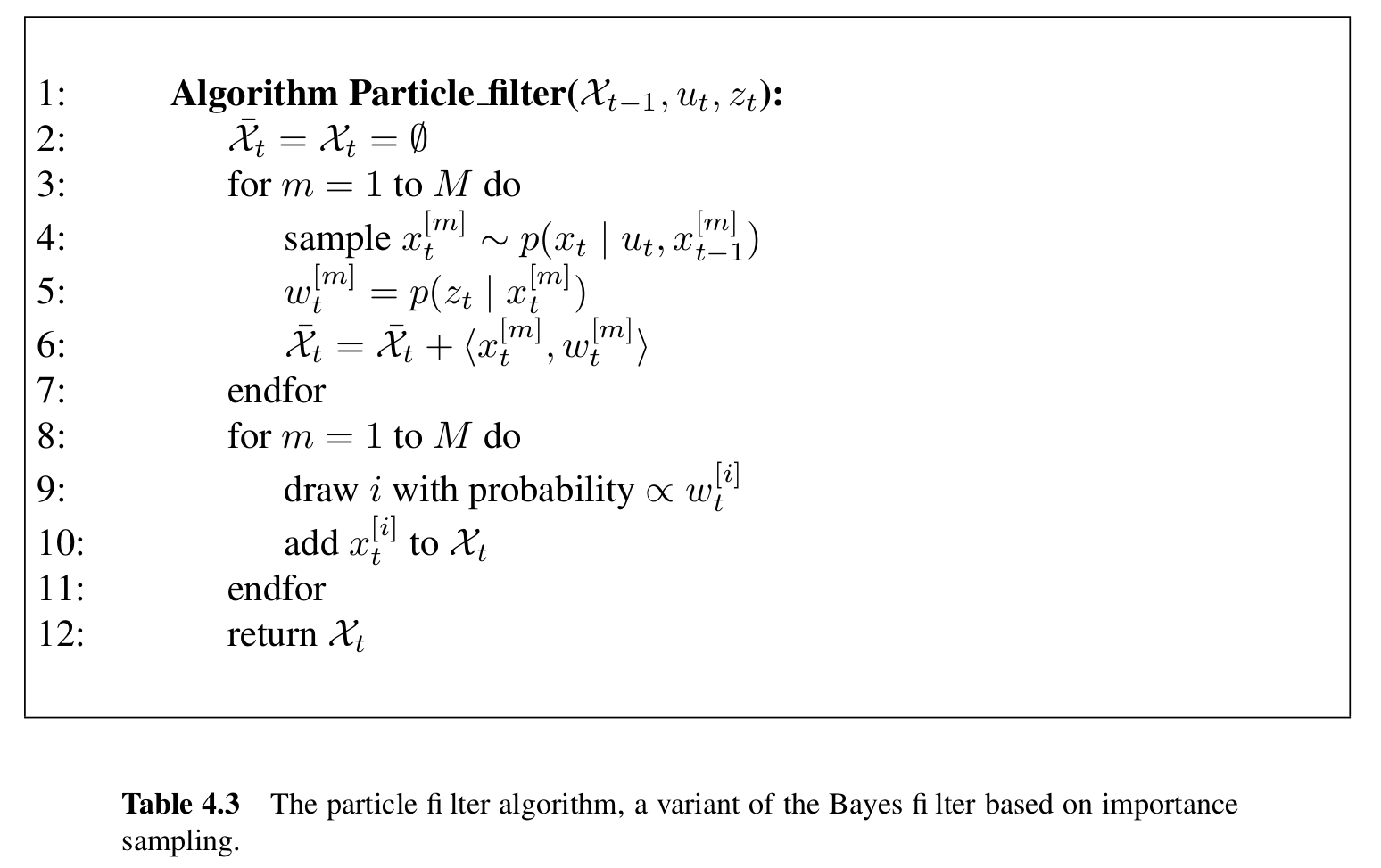算法4.3中第3、4、5行相当于循环取一个粒子求他们的权重，这个权重需要把每个粒子与所有的激光进行坐标变换（激光距离信息转换到激光坐标系下，然后把粒子转换带全局坐标系下），然后去从似然地图中得到似然，最后累加所以激光得到的似然获得该粒子的权重。
第6行没懂什么意思。
第8-11行是粒子滤波最重要的重采样，这里的重采样会出现机器人不动，而还在进行重采样，造成失去低概率区域的许多样本，最后造成很差的定位效果。
测量更新代码：
/*测量更新，中文概率机器人第4章 P74 程序4.3*/
void Localizer::measurementUpdate ( const sensor_msgs::LaserScanConstPtr& scan )
{
if ( !is_init_ )
{
return;
}

/* 获取激光的信息 */
const double& ang_min = scan->angle_min;
const double& ang_max = scan->angle_max;
const double& ang_inc = scan->angle_increment;
const double& range_max = scan->range_max;
const double& range_min = scan->range_min;
/*求每个粒子的权重*/
for ( size_t np = 0; np < nParticles_; np ++ )
{
Particle& pt = particles_.at ( np );
/* for every laser beam */
/* 对每个激光返回的点求似然，然后累加得到这个粒子的总似然做权重*/
for ( size_t i = 0; i < scan->ranges.size(); i ++ )
{
/* 获取当前beam的距离 */
const double& R = scan->ranges.at ( i );//常量引用
if ( R > range_max || R < range_min )
continue;

double angle = ang_inc * i + ang_min;
double cangle = cos ( angle );
double sangle = sin ( angle );
Eigen::Vector2d p_l (
R * cangle,
R* sangle
); //激光数据在雷达的坐标系下的坐标

/* 转换到世界坐标系下 概率机器人第6章 P128 公式6.32，这里相当于转换到每个粒子的坐标系下去匹配似然*/
Pose2d laser_pose = pt.pose_ * robot_->T_r_l_;//用运算符重载 * 计算雷达的全局坐标和角度
Eigen::Vector2d p_w = laser_pose * p_l;//用运算符重载 * 计算激光点在全局坐标系下的坐标

/* 更新weight */
double likelihood = measurement_model_->getGridLikelihood ( p_w ( 0 ), p_w ( 1 ) );//从初始化的似然地图上获取似然值

/* 整合多个激光beam用的加法， 用乘法收敛的太快 */
pt.weight_ += likelihood;
}// for every laser beam
} // for every particle

/* 权重归一化 */
normalization();

/* TODO 这里最好使用书上的Argument 的重采样 + KLD重采样方法.
*重采样频率太高会导致 粒子迅速退化 */
static int cnt = -1;
if  ( (cnt ++)  % 10 == 0 ) //减少重采样的次数
reSample();    //低方差重采样
}

2.1.3 重采样代码解读 void reSample();
概率机器人P78 程序4.4重采样算法：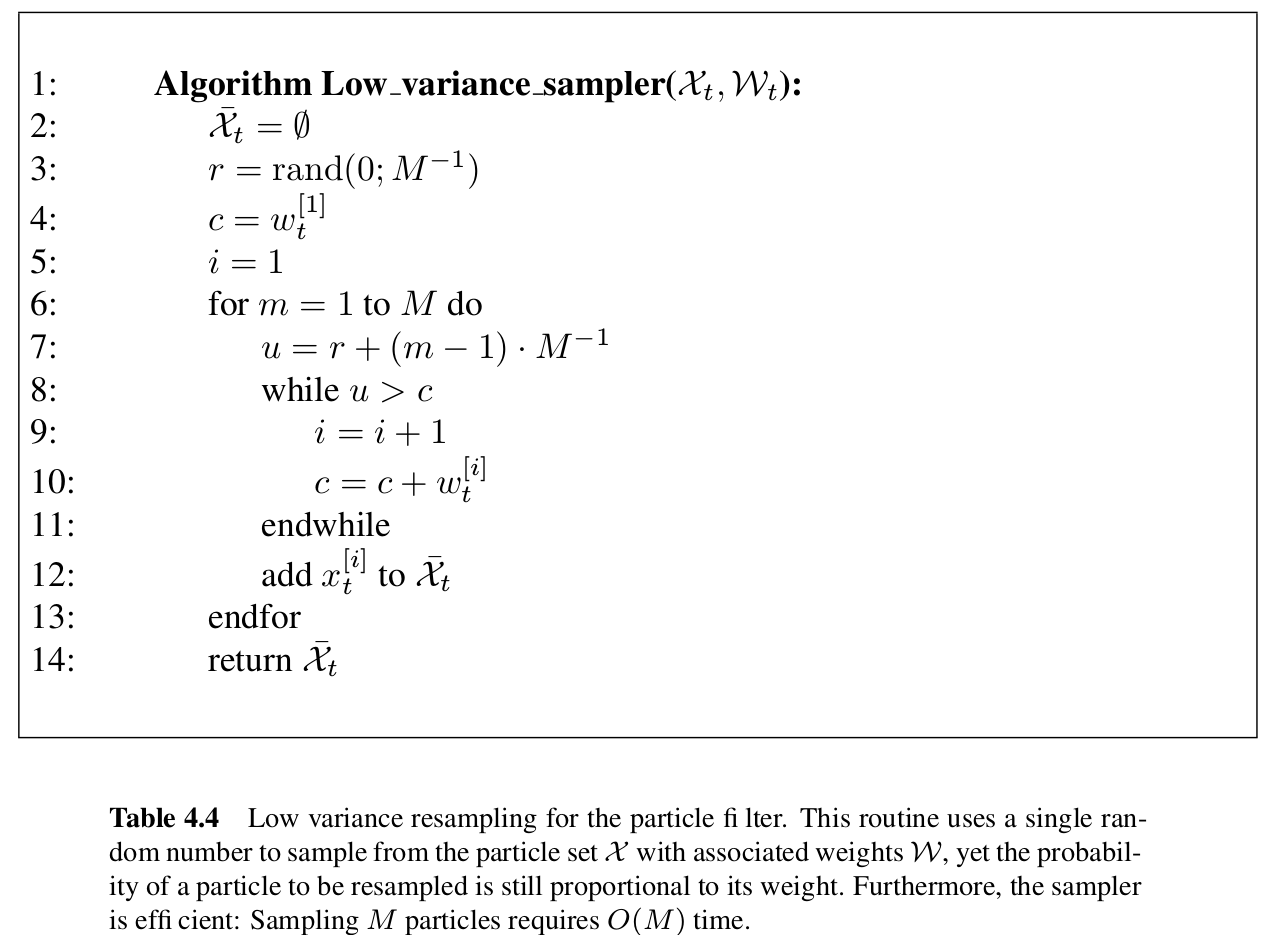算法4.4是低方差重采样算法，该算法可以更有序的形式覆盖样本空间、避免了样本丢失和复杂度为O(M)。
那么如何来理解重采样算法4.4呢？
整个重采样过程有一个0-

M

−

1

M^{-1}

的随机数，如算法第3行所示。
算法中while循环完成两个任务：计算等式右边的和;检查i是否是第一个粒子的索引，对应的权值是否超过U。在第12行进行选择。
第6-12行请看下图理解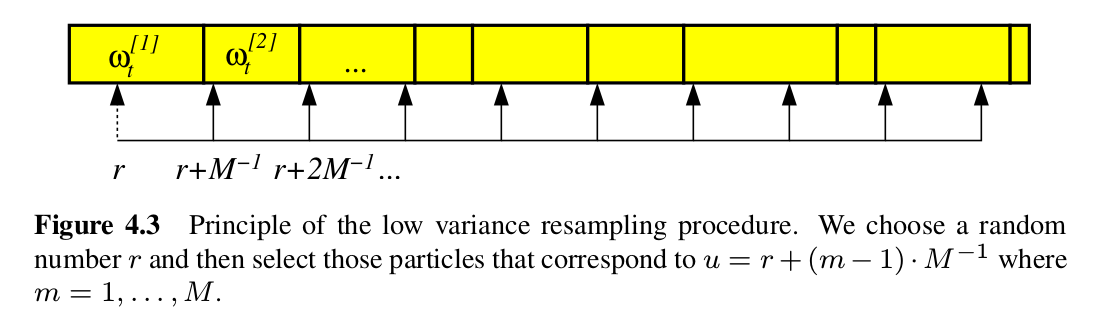上图中每个箭头的间距都为

M

−

1

M^{-1}

，如果粒子的累计权重>此刻的U，就把该粒子加入新的粒子集，该部分进行了重采样。
重采样代码：
/*低方差重采样的理解可以看概率机器人第四章 P82 理解，算法在P78 程序4.4*/
void Localizer::reSample()
{
if ( !is_init_ )
return;

/* 重采样 ，可见概率机器人第4章粒子滤波的低方差重采样算法，程序4.4,p78*/
std::vector<Particle> new_particles;
/*
RNG可以产生3种随机数
RNG(int seed)         使用种子seed产生一个64位随机整数，默认-1
RNG::uniform( )      产生一个均匀分布的随机数
RNG::gaussian( )    产生一个高斯分布的随机数
*/
cv::RNG rng ( cv::getTickCount() );
long double inc = 1.0 / nParticles_;
long double r = rng.uniform ( 0.0, inc );//从0,inc随机取数，第3行
long double c = particles_.at ( 0 ).weight_;//取第一个粒子的权重，第四行
int i = 0;

/*第6-12行*/
for ( size_t m = 0; m < nParticles_; m ++ )
{
long double U = r + m * inc;//通过随机的r加上均值概率和
while ( U > c )//该循环完成两个任务：计算该等式右边的和;检测i是否是第一个粒子的索引，对应的权值和是否超过U。
{
i = i+1;
c = c + particles_.at ( i ).weight_;//粒子权重累加
}
particles_.at ( i ).weight_ = inc;//把概率大的粒子的权重变为1/M

/* 在概率大的粒子上进行采样，直到采满m个，新采样的粒子加了高斯，稍微增加一下多样性，新粒子的权重都为1/M */
Particle new_pt = particles_.at(i);
new_pt.pose_.x_ += rng.gaussian(0.02);
new_pt.pose_.y_ += rng.gaussian(0.02);
new_pt.pose_.theta_ += rng.gaussian(0.02);//产生均值为0,方差为0.02的高斯随机数
Pose2d::NormAngle(new_pt.pose_.theta_);
new_particles.push_back ( new_pt );
}
particles_ = new_particles;//更新之前的粒子群
}

2.2 measurement_model.cc代码解读
该代码主要用于求似然，用于粒子的权重更新。
该算法在中文版概率机器人P130 程序6.3。
似然值计算算法如下：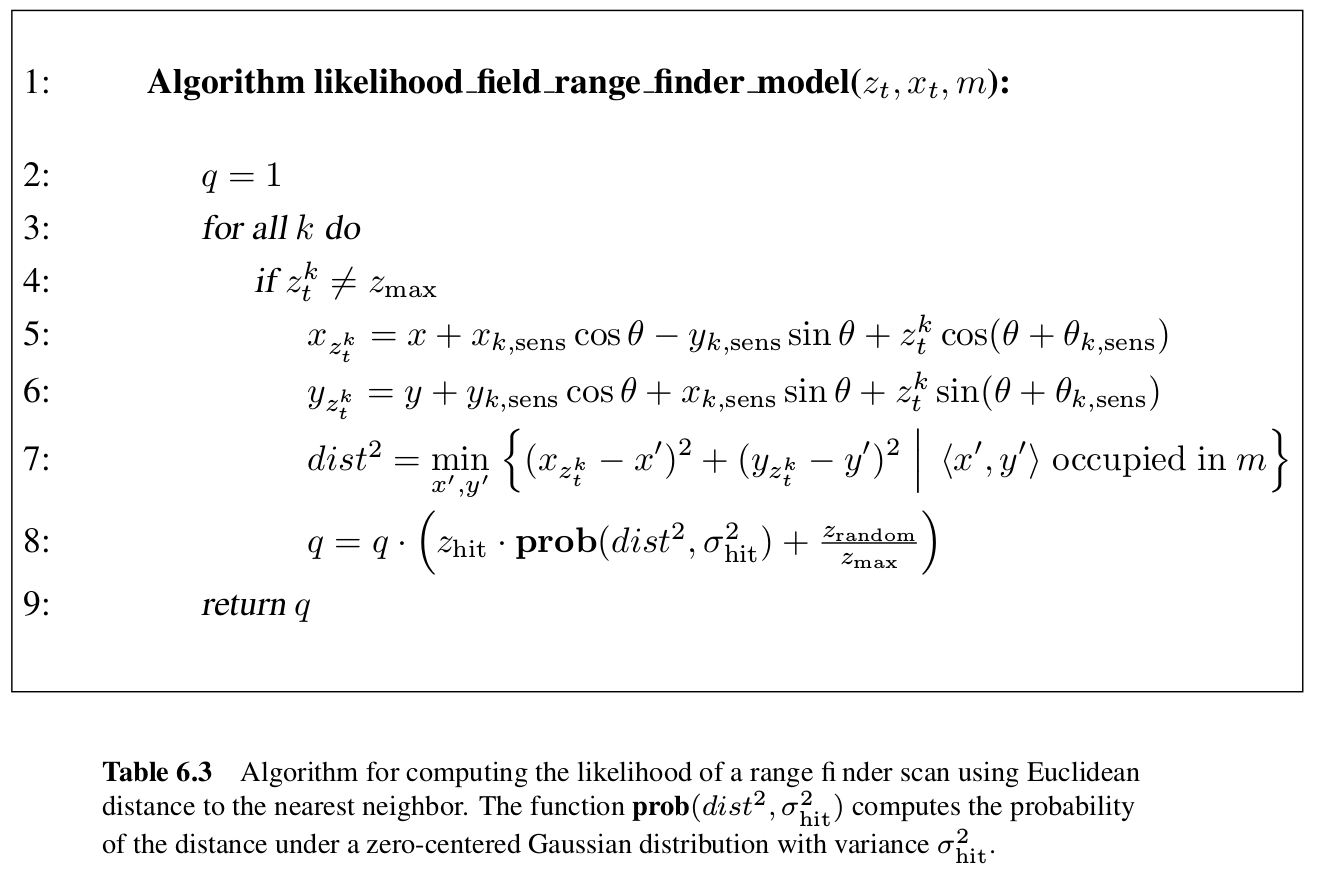算法6.3和下面的程序有些许不同
算法6.3的第4行用于检查传感器读书是否为最大距离读数，如果是则舍弃。
第5-6行把雷达测得的距离信息结合粒子位置转换到全局地图坐标系。
第7行，根据已有地图，把全局坐标系下测得的障碍为位置信息与已有地图中最近障碍物的算欧式距离。
第8行通过一个正态分布和一个均匀分布混合得到似然结果。
而下面代码中，直接定义一个似然域地图，通过提前计算好地图上每个位置的似然值，然后后面直接通过粒子直接在该地图上查询得到似然值。
在程序在通过求似然域地图和粒子信息做比较获取似然值，代码如下：
/* 中文版概率机器人第6章 P130 程序6.3 */
MeasurementModel::MeasurementModel ( GridMap* map, const double& sigma, const double& rand ):
map_(map), sigma_(sigma), rand_(rand)
{
/* 初始化似然域模型, 预先计算的似然域模型的格子大小比占有栅格小1倍*/
size_x_ = 2 * map->size_x_;
size_y_ = 2 * map->size_y_;
init_x_ = 2 * map->init_x_;
init_y_ = 2 * map->init_y_;
cell_size_ = 0.5 * map->cell_size_;

likelihood_data_.resize(size_x_ , size_y_); //这个似然域地图，用于后面激光点打到的位置的似然值进行查表
likelihood_data_.setZero();

/* 构造障碍物的KD-Tree，用到了PCL库函数 */
pcl::PointCloud<pcl::PointXY>::Ptr cloud (new pcl::PointCloud<pcl::PointXY>);//pcl::PointXY储存2维点云

for(int i = 0; i < map->size_x_;  i ++)
for(int j = 0; j < map->size_y_; j ++)
{
if(map->bel_data_(i, j) == 1.0) //是障碍物就加到KD树中
{
pcl::PointXY pt;
pt.x = (i - map->init_x_) * map->cell_size_;//获取障碍物到世界坐标原点的相对距离
pt.y = (j - map->init_y_) * map->cell_size_;
cloud->push_back(pt);
}
}
kd_tree_.setInputCloud(cloud);

/* 对每个格子计算likelihood */
for(double i = 0; i < size_x_; i += 0.9)
for(double j = 0; j < size_y_; j +=0.9)
{
/* 计算double x, y */
double x = ( i - init_x_)* cell_size_;
double y = ( j- init_y_ ) * cell_size_;
double likelihood =  likelihoodFieldRangFinderModel(x, y);
setGridLikelihood(x, y, likelihood);
}
}

double MeasurementModel::likelihoodFieldRangFinderModel ( const double& x, const double& y )
{
/* 找到最近的距离 */
pcl::PointXY search_point;
search_point.x = x;
search_point.y = y;
std::vector<int> k_indices;
std::vector<float> k_sqr_distances;
int nFound =  kd_tree_.nearestKSearch(search_point, 1, k_indices, k_sqr_distances);//1代表最近的一个目标，获取离x,y最近的障碍物的欧式距离
double dist = k_sqr_distances.at(0);

/* 高斯 + random */
return gaussion(0.0, sigma_, dist) + rand_;/******使用高斯概率，均值为0,越靠近0概率越大，返回值越大******/
}

double MeasurementModel::gaussion ( const double& mu, const double& sigma, double x)/******返回正态分布的概率******/
{
return (1.0 / (sqrt( 2 * PI ) * sigma) ) * exp( -0.5 * (x-mu) * (x-mu) / (sigma* sigma) );
}

bool MeasurementModel::getIdx ( const double& x, const double& y, Eigen::Vector2i& idx )/*判断该点是否在似然域地图内，返回坐标*/
{
int xidx = cvFloor( x / cell_size_ ) + init_x_;//cvFloor 返回不大于参数的最大整数值
int yidx  = cvFloor( y /cell_size_ )+ init_y_;

if((xidx < 0) || (yidx < 0) || (xidx >= size_x_) || (yidx >= size_y_))
return false;
idx << xidx , yidx;//把坐标返回给idx
return true;
}

bool MeasurementModel::setGridLikelihood ( const double& x, const double& y, const double& likelihood )
{
Eigen::Vector2i idx;
if(!getIdx(x, y, idx))//获取世界似然地图的坐标
return false;
likelihood_data_(idx(0), idx(1)) = likelihood;//在地图对应的位置上记录打到障碍物的概率（似然）
return true;
}


上面代码中运用了PCL点云库（可参考点云库PCL从入门到精通 第4章 P100）来**构造KD-tree**作为存放障碍物位置的数据结构，通过kd_tree_.nearestKSearch(search_point, 1, k_indices, k_sqr_distances)函数，可以查找出距离search_point点最近障碍物，以及计算出他们间的欧式距离，1代表只需找最近的1个点，k_indices代表在KD-tree中的坐标，k_sqr_distances代表计算出来的欧式距离。
2.3 pf_localization_node.cc代码解读
这是整个代码的主函数，主要用于参数读入，话题的订阅和发布
GridMap* g_map;
ros::Subscriber g_odom_suber;
ros::Publisher g_map_puber, g_particles_puber;
Localizer* g_localizer;

void odometryCallback ( const nav_msgs::OdometryConstPtr& odom );
void laserCallback ( const sensor_msgs::LaserScanConstPtr& scan );

int main ( int argc, char **argv )
{
/***** 初始化ROS *****/
ros::init ( argc, argv, "PF_Localization" );
ros::NodeHandle nh;

/* 加载地图 */
std::string map_img_dir, map_cfg_dir;
int initx, inity;
double cell_size;
/* TODO 错误处理 */
nh.getParam ( "/pf_localization_node/map_dir", map_img_dir );//获取地图的图片地址
nh.getParam ( "/pf_localization_node/map/initx", initx );//世界地图的原点坐标
nh.getParam ( "/pf_localization_node/map/inity", inity );
nh.getParam ( "/pf_localization_node/map/cell_size", cell_size );//获取地图分辨率
GridMap* map = new GridMap();//定义一个地图类用于存放地图的数据
map->loadMap ( map_img_dir, initx, inity, cell_size );//导入地图
g_map = map;

/* 初始化机器人 */
Pose2d T_r_l;
double x, y, theta;
/* TODO 错误处理 */
nh.getParam ( "/pf_localization_node/robot_laser/x", x );
nh.getParam ( "/pf_localization_node/robot_laser/y", y );
nh.getParam ( "/pf_localization_node/robot_laser/theta", theta );
T_r_l = Pose2d ( x, y, theta );//雷达的位置
Robot* robot = new Robot ( 0.0, 0.0, 0.0, T_r_l );//初始化机器人的位置和雷达相对于机器人的位置

/* 初始化运动模型 */
double a1, a2, a3, a4;//获取机器人运动误差系数
nh.getParam ( "/pf_localization_node/motion_model/alpha1", a1 );
nh.getParam ( "/pf_localization_node/motion_model/alpha2", a2 );
nh.getParam ( "/pf_localization_node/motion_model/alpha3", a3 );
nh.getParam ( "/pf_localization_node/motion_model/alpha4", a4 );
MotionModel* motion_model = new MotionModel ( a1, a2, a3, a4 );//实例化一个对象用于存放和计算机器人的运动模型

/* 初始化观测模型 */
double sigma, rand;
nh.getParam ( "/pf_localization_node/measurement_model/sigma", sigma );
nh.getParam ( "/pf_localization_node/measurement_model/rand", rand );
std::cout << "\n\n正在计算似然域模型, 请稍后......\n\n";
MeasurementModel* measurement_model = new MeasurementModel ( map, sigma, rand );//实例化一个对象存放地图每个位置的似然数据
std::cout << "\n\n似然域模型计算完毕.  开始接收数据.\n\n";

/* 初始化定位器 */
int n_particles;
nh.getParam ( "/pf_localization_node/particle_filter/n_particles", n_particles );//获取需要多少粒子数
g_localizer = new Localizer ( robot, map, motion_model, measurement_model, n_particles );//初始化例子集合
std::cout<<"\n\n初始化粒子群\n\n";

/* 初始Topic */
std::string odom_topic_;
nh.getParam( "/pf_localization_node/robot/odom_topic", odom_topic_);
g_odom_suber = nh.subscribe ( odom_topic_, 1, odometryCallback );//P3DX机器人的里程计话题为/RosAria/pose,这里为：/mbot/odometry
ros::Subscriber laser_suber = nh.subscribe ( "/scan", 1, laserCallback );//订阅雷达信息
g_map_puber = nh.advertise<nav_msgs::OccupancyGrid> ( "pf_localization/grid_map", 1 );//发布栅格地图
g_particles_puber = nh.advertise<geometry_msgs::PoseArray> ( "pf_localization/particles", 1 );//发布粒子的信息
ros::spin();
}

void odometryCallback ( const nav_msgs::OdometryConstPtr& odom )
{
/* 获取机器人姿态 */
double x = odom->pose.pose.position.x;//获取机器人的坐标和角度
double y = odom->pose.pose.position.y;
double theta = tf::getYaw ( odom->pose.pose.orientation );
Pose2d rpose ( x, y, theta );

/* 运动更新 */
g_localizer->motionUpdate ( rpose );

/* 发布粒子 */
geometry_msgs::PoseArray pose_array;
g_localizer->particles2RosPoseArray ( pose_array );//把Pose2d类型的数据变为ros话题传输数据类型geometry_msgs::PoseArray
g_particles_puber.publish ( pose_array );

}

void laserCallback ( const sensor_msgs::LaserScanConstPtr& scan )
{
/* 测量更新 */
g_localizer->measurementUpdate ( scan );

/* 低频发布地图 */  //按道理来讲只需要发布一次就行了呀。可能为了用于slam中同时建图定位出现的地图更新
static int cnt = -1;
if  ( (cnt ++)  % 100 == 0 )
{
nav_msgs::OccupancyGrid occ_map;
g_map->toRosOccGridMap ( "odom", occ_map );
g_map_puber.publish ( occ_map );
}
}

其中nh.getParam用于获取参数配置文件的参数。
3. 运行自己地图需要修改的部分
3.1 参数配置文件
参数的配置文件default.yaml如下：
# 地图的基本参数，建图的时候获得
map:
sizex: 10 #地图的大小
sizey: 10
initx: -5 #地图的坐标原点
inity: -5
cell_size: 0.05 # 分辨率
frame_id: odom

sensor_model:
P_occ: 0.6
P_free: 0.4
P_prior: 0.5
# 机器人的固有参数
robot:
kl: 2.31249273072714e-06
kr: 2.30439760302837e-06
b: 0.719561485930414
odom_topic: /mbot/odometry #机器人发布的里程计话题，会根据不同机器人发布的话题不同而不同，需修改
# 雷达和机器人坐标系下的位置，用于tf变换，根据雷达放在机器人身上的位置不同而不同
robot_laser:
x: 0.26
y: 0.0
theta: 0.0
# 机器人运动的噪音系数
motion_model:
alpha1: 0.15
alpha2: 0.15
alpha3: 0.15
alpha4: 0.15
# 高斯的参数
measurement_model:
sigma: 0.2 # 方差
rand: 0.01 # 偏置
# 粒子滤波的粒子数，粒子滤波的关键
particle_filter:
n_particles: 1000

3.2 pf_localization.launch文件配置
<node pkg="particle_filter_localization" type="odometry" name="odometry" output="screen" clear_params="true">
<!--加载参数配置文件-->
<rosparam file="$(find particle_filter_localization)/config/default.yaml" command="load" /> </node> <node pkg="particle_filter_localization" type="pf_localization_node" name="pf_localization_node" output="screen" clear_params="true"> <rosparam file="$(find particle_filter_localization)/config/default.yaml" command="load" />

<!--加载地图的png图片-->
<param name="map_dir" value="$(find particle_filter_localization)/map/map.png" /> </node> <node pkg="rviz" type="rviz" name="rviz" output="screen" args="-d$(find particle_filter_localization)/rviz/default.rviz" required="true">
</node>

</launch>

展开全文自动驾驶
• 针对粒子滤波在跟踪非线性状态突变系统的隐状态时,因粒子贫化导致估计精度下降的问题,提出一种基于Student's t分布的自适应重采样粒子滤波算法.首先,将Student's t分布作为采样尺度转移方程,再自适应地将粒子依据...
• 针对粒子滤波存在的重要性密度函数难以选取和可能出现粒子退化的问题，在吸收平方根滤波、自适应滤波和粒子滤波优点的基础上，提出了一种新的UPF算法。该算法由UKF算法得到重要性密度函数，通过自适应因子实时控制...
• 包括基于重要性密度函数选择的粒子滤波算法、基于重采样技术的粒子滤波算法、基于智能优化思想的粒子滤波算法、自适应粒子滤波算法、流形粒子滤波算法等，并将粒子滤波算法应用于机动目标跟踪、语音增强、传感器故障...
• 该算法结合了均值漂移算法运算效率高的和粒子滤波算法能够有效处理遮挡情况的特点。实验表明该算法可以高效地跟踪多目标、准确判断目标的出现和消失，并能够解决多目标冲突、合并和分离等问题。
• 针对当前粒子滤波权值退化问题以及精度与时耗的矛盾, 提出了一种新的高精度自适应粒子滤波算法。该算法综合考虑优选建议分布函数和重采样两种并行改进滤波性能的方法：首先, 在积分卡尔曼滤波QKF的基础上引入修正...
• 是一种基于粒子群优化算法思想的组合自适应滤波算法，关于粒子群的一种滤波算法
• 基于粒子群和麻雀搜索的LMS自适应滤波算法 文章目录基于粒子群和麻雀搜索的LMS自适应滤波算法1.LMS 自适应滤波算法2.自适应滤波在降噪中的应用3.粒子群算法对LMS滤波算法的改进4.PSO—LMS算法实验5.SSA—LMS算法实验...
基于粒子群和麻雀搜索的LMS自适应滤波算法

文章目录
基于粒子群和麻雀搜索的LMS自适应滤波算法1.LMS 自适应滤波算法2.自适应滤波在降噪中的应用3.粒子群算法对LMS滤波算法的改进4.PSO—LMS算法实验5.SSA—LMS算法实验6.参考文献7.Matlab代码

摘要：在自适应滤波算法中，LMS算法是最常用的算法之一，因为具备结构简单，易于实现，性能稳定，计算复杂度低等特
点。然而， LMS算法也存在缺点，比如，收敛速度较慢，收敛精度低的问题，这就影响LMS算法在收敛性要求较高的领域中的
应用。使用粒子群算法和麻雀搜索算法对 LMS算法进行改进，可以将 LMS滤波设计变成对 LMS滤波参数优化的问题，利用粒子群算法的优化能力，使得滤波参数得到全局最优解。以此可以提高 LMS滤波算法的收敛性能，从而提高滤波性能。

1.LMS 自适应滤波算法
LMS滤波器的基本结构，如图1所示。根据如图1所示，该图为LMS滤波器的基本原理框图：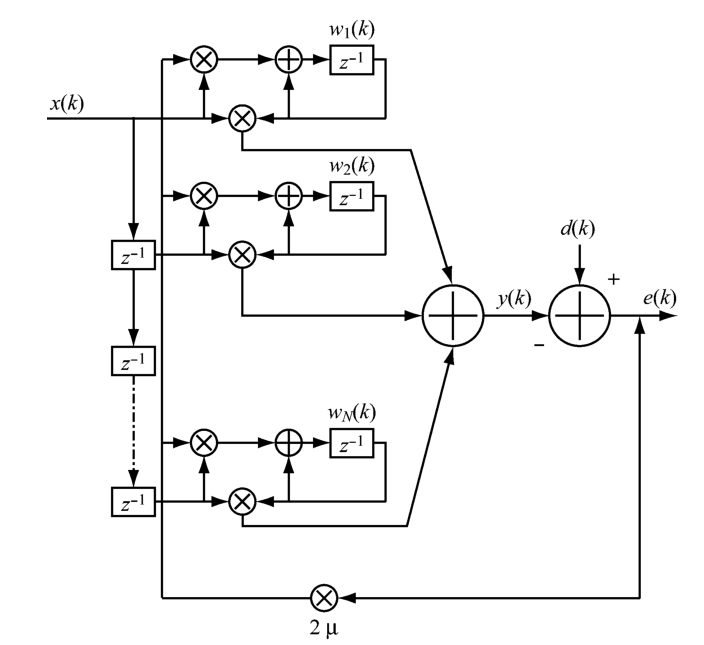图1.LMS滤波器基本结构

初始化时，如式（1）：

w

(

0

)

=

w

(

0

)

=

[

000...0

]

T

w(0)=w(0)=[0 0 0 ... 0]^T

当 ｋ ≥０ 时，如式(２)、式(３)

e

(

k

)

=

d

(

k

)

−

x

T

(

k

)

w

(

k

)

(2)

e(k) = d(k) - x^T(k)w(k) \tag{2}

w

(

k

+

1

)

=

w

(

k

)

−

2

u

e

(

k

)

x

(

k

)

(3)

w(k+1) =w(k)-2ue(k)x(k)\tag{3}

其中，(k)为瞬时的误差，u为收敛因子，e(k)是误差信号，w(k)为的滤波器系数。按照梯度特性,w(k)在每次迭代运算中会自动调整，逐步是均值

E

[

e

2

(

k

)

]

E[e^2(k)]

最小化，

E

[

e

2

(

k

)

]

E[e^2(k)]

就是最小均方差。
2.自适应滤波在降噪中的应用
当自适应滤波被应用在降噪应用中是，它的结构框图，如图 2 所示.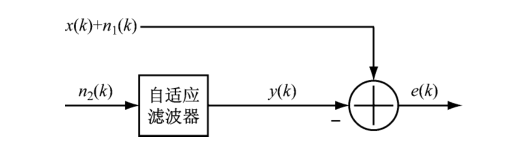图2.信号降噪结构

与看见的自适应滤波器结构是不同的。信号

x

(

k

)

x(k)

受到噪声

n

1

(

k

)

n_1(k)

的影响。而信号

n

2

(

k

)

n_2(k)

是与噪声相关的信号，它可以被测量到的信号。

n

2

(

k

)

n_2(k)

也作为自适应滤波器的输入信号，受到干扰的信号

x

(

k

)

+

n

1

(

k

)

x(k) + n_1(k)

作为期望信号。
输出信号

y

(

k

)

y(k)

与输入信号

n

2

(

k

)

n_2(k)

的数学关系式根据图 １是式(4)

y

(

k

)

=

∑

l

=

0

N

w

l

n

2

(

k

−

l

)

(4)

y(k) = \sum_{l=0}^{N}w_ln_2(k-l)\tag{4}

按照均方误差方程，可以得到式(5)

E

[

e

2

(

k

)

]

=

E

[

x

2

(

k

)

]

+

E

[

n

1

(

k

)

−

y

(

k

)

]

2

(5)

E[e^2(k)] = E[x^2(k)] + E{[n_1(k) - y(k)]^2}\tag{5}

假如

x

(

k

)

x(k)

与

n

1

(

k

)

n_1(k)

和

n

2

(

k

)

n_2(k)

无关，那么该函数的最小

M

S

E

MSE

为式(6)

ξ

m

i

n

=

E

[

e

2

(

k

)

]

=

E

[

x

2

(

k

)

]

(6)

\xi_{min} = E[e^2(k)] = E[x^2(k)]\tag{6}

其中

x

(

k

)

x(k)

就是我们滤波所要得到的信号。
3.粒子群算法对LMS滤波算法的改进
式２和式３所示的更新方程是LMS算法的最重要的工作步骤。根据梯度特性

E

[

e

2

(

k

)

]

E[e^2(k)]

会不断趋于最小均方差。其中。从式2能够推倒出下式(7)。

e

(

k

+

1

)

=

d

(

k

+

1

)

+

x

T

(

k

+

1

)

[

w

(

k

)

−

2

u

e

(

k

)

x

(

k

)

]

(7)

e(k+1) = d(k+1) + x^T(k+1)[w(k)-2ue(k)x(k)]\tag{7}

e

(

k

)

e(k)

是瞬时误差，根据式 7 所示，收敛因子2u决定了

E

[

e

2

(

k

)

]

E[e^2(k)]

的最小值。许多研究都是针对2u ，通过动态调整2u使得 值逐

E

[

e

2

(

k

)

]

E[e^2(k)]

步达到最小，从而提高收敛性。而文献则使用粒子群算法优化能力，使得

E

[

e

2

(

k

)

]

E[e^2(k)]

每次迭代中做到最小化，实现 LMS 滤波的最优收敛效果，从而提升滤波降噪能力。
首先将收敛因子u设为搜索空间内的粒子，那么对 u的调整操作就转换为寻找粒子在空间的最优位置。
根据式（7），本文设定适应度函数，如下式（8）

F

=

m

i

n

(

e

(

k

+

1

)

)

(8)

F = min(e(k+1)) \tag{8}

该适应度函数能够实现瞬时误差的最小化，从而是最小均方差 MSE达到最小。
4.PSO—LMS算法实验
利用正弦信号加噪声生成模拟数据，数据如下图所示：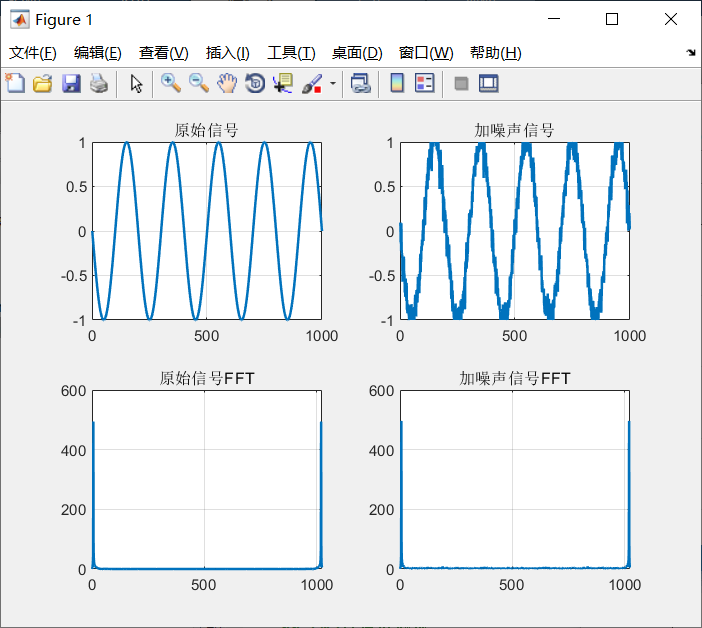经过原始LSM和PSO-LSM滤波后的对比图如下并且利用绝对误差和做为评价标准：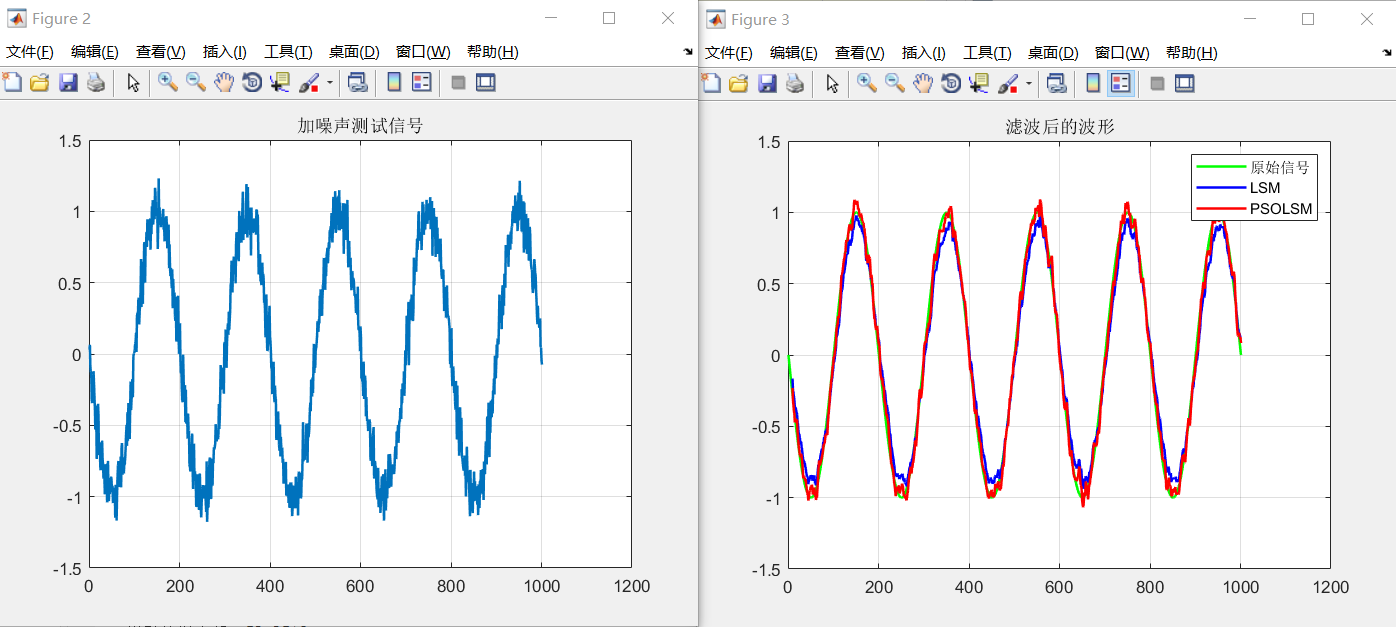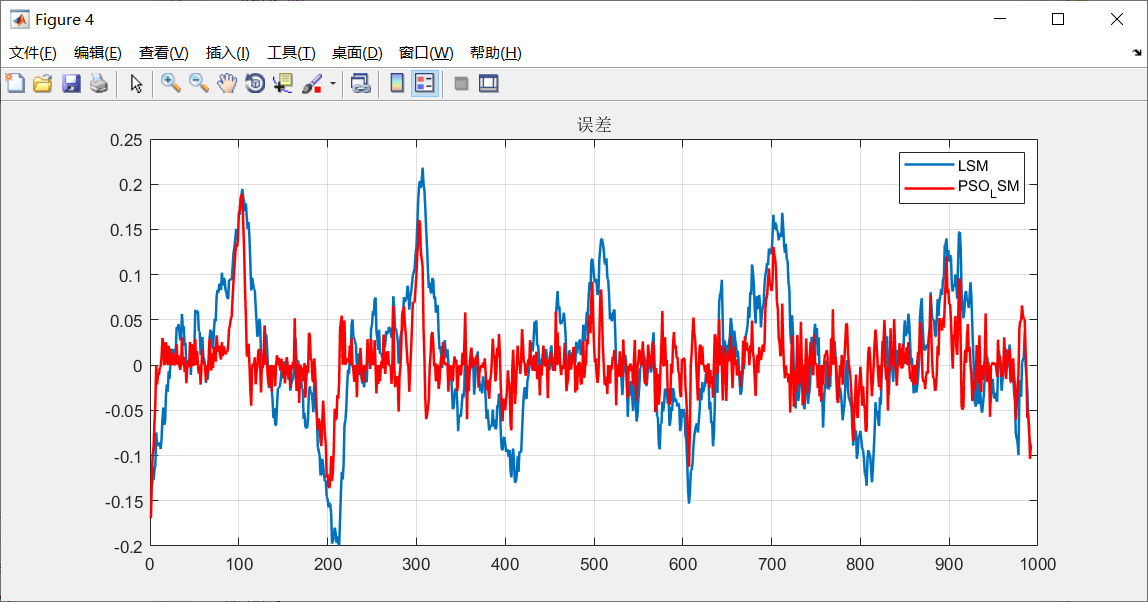从结果上来看改进后的LMS明显优于基础LMS滤波，滤波后的信号更接近原始信号，误差更小。
5.SSA—LMS算法实验
根据同样的原理利用麻雀搜索算法对LMS滤波算法进行改进
麻雀搜索算法的具体原理参考博客：https://blog.csdn.net/u011835903/article/details/108830958。
测试结果如下：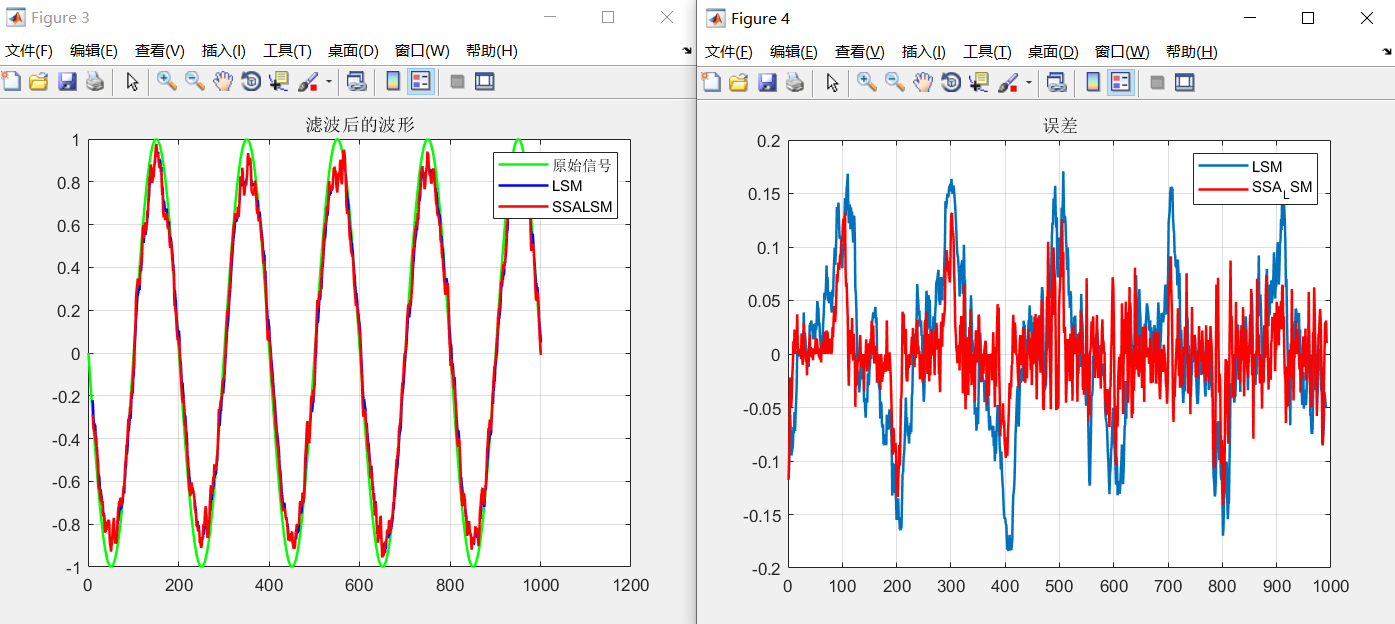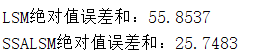可以看到麻雀搜索算法对LMS滤波的提升仍然是比较明显的。
同时运行PSO-LMS和SSA-LMS得到如下结果：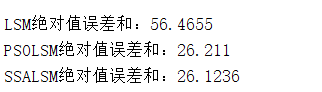可以看到SSA-LMS的效果更好，误差和更小。
6.参考文献
赵轶骁,汪镭.基于粒子群的LMS算法在信号滤波降噪中的应用[J].微型电脑应用,2017,33(09):71-74.
7.Matlab代码
基于粒子群的LMS滤波算法 基于麻雀搜索算法的LMS滤波算法 个人资料介绍`
展开全文python 深度学习
• 将状态转移先验分布和观测似然分布相结合，提出一种基于自适应退火参数优化混合建议分布的粒子滤波算法。根据当前最新的观测信息，以退火参数因子调控混合建议分布中状态转移先验分布与似然建议分布的混合率。在混合...
• 为了改进粒子滤波算法的性能，这里研究了一种粒子滤波算法改进策略。该粒子滤波算法改进策略包括四部分：首先，采用了结合退火参数的混合建议分布，以考虑当前观测测量值的最新信息；接着，基于有效样本大小确定...
• 通过对粒子滤波算法中建议分布与重采样2种改进技术分析,提出了一种粒子滤波自适应优化算法.首先,基于退火参数自适应优化混合建议分布,以改进建议分布的采样范围.然后,在基于有效样本大小的自适应重采样技术之上,借助...
• 针对基于Kalman滤波的PSO算法在设计与应用过程中存在的不足,提出了基于自适应Kalman滤波的改进PSO算法。利用粒子群状态空间Markov链模型,建立粒子群系统状态方程;采用粒子的速度和位置作为观测量,构建观测方程;引入...
• 针对基于粒子群优化的粒子滤波(PSO-PF) 算法精度不高, 实时性差, 难以满足雷达机动目标跟踪的需求, 提 出一种基于动态邻域自适应粒子群优化的粒子滤波(DPSO-PF) 算法. 该算法可以动态调整粒子邻域环境, 其中每个...
• 基于加权颜色直方图与梯度方向直方图自适应融合的粒子滤波跟踪算法 matlab仿真 直升机目标跟踪 例子，使用多特征自适应融合的 粒子滤波方法，注意这是32位系统版本，64位需要重新对c语言进行编译。
• 基于特征融合的粒子滤波算法可以将多个不同的特征进行融合，增强跟踪系统鲁棒性，但是现有的算法存在着特征显著性差，算法实时性不强以及融合策略不具备通用性的缺点。针对上述问题提出了一种适用于前车追踪系统的...
• 在粒子滤波的基础上融合扩展卡尔曼滤波算法，融合后的算法在计算提议概率密度分布时...将此改进粒子滤波算法在“当前”统计模型框架下进行机动目标自适应跟踪。仿真实验验证了该种方法对机动目标的良好自适应跟踪性能。...